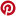# Types of Polygons

Related Topics: More about Polygons
Polygon Games
More Grade 3 Lessons
More Geometry Lessons

Videos, worksheets, stories and songs to teach Grade 3 students the types of polygons: Triangles, Quadrilaterals, Pentagon, Hexagon, Heptagon, Octagon, Nonagon, Decagon.

A polygon is a closed plane (2-D) figure having three or more straight sides that intersect only at the vertices. Polygons have the same number of sides as vertices. Polygons are identified by their number of sides.

A regular polygon has equal sides and equal angles. An irregular polygon is a polygon whose sides are not all the same length. A circle is not a polygon because it does not have straight line segments.

The following table gives the names of some polygons and examples of regular polygons and irregular polygons. Scroll down the page for more examples, songs and explanations about polygons.Polygon Song by Peter Weatherall

I am a parallelogram song by Peter Weatherall Learn the definition of polygon - a very important shape in geometry
When a polygon has a small number of sides, there is a word you use instead of "polygon".
We teach you the names of polygons with 3 to 10 sides. In this catchy song, learn to identify polygons: from triangles to decagons Names of Basic Shapes Naming Polygons
Math Lesson about different types of polygons. Some polygons include triangle, quadrilateral, pentagon, hexagon, heptagon, octagon, nonagon, and decagon.

Try the free Mathway calculator and problem solver below to practice various math topics. Try the given examples, or type in your own problem and check your answer with the step-by-step explanations.We welcome your feedback, comments and questions about this site or page. Please submit your feedback or enquiries via our Feedback page.